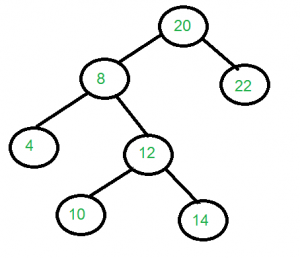# Construct a tree from Inorder and Level order traversals | Set 2

Given inorder and level-order traversals of a Binary Tree, construct the Binary Tree. Following is an example to illustrate the problem.

Examples:

```Input: Two arrays that represent Inorder
and level order traversals of a
Binary Tree
in[]    = {4, 8, 10, 12, 14, 20, 22};
level[] = {20, 8, 22, 4, 12, 10, 14};

Output: Construct the tree represented
by the two arrays.
For the above two arrays, the
constructed tree is shown.```

## Recommended: Please solve it on “PRACTICE” first, before moving on to the solution.

We have discussed a solution in below post that works in O(N^3)
Construct a tree from Inorder and Level order traversals | Set 1

Approach : Following algorithm uses O(N^2) time complexity to solve the above problem using the unordered_set data structure in c++ (basically making a hash-table) to put the values of left subtree of the current root and later and we will check in O(1) complexity to find if the current levelOrder node is part of left subtree or not.

If it is the part of left subtree then add in one lLevel arrray for left other wise add it to rLevel array for right subtree.

Below is the c++ implementation with the above idea

 `/* program to construct tree using inorder  ` `   ``and levelorder traversals */` `#include ` `#include ` `using` `namespace` `std; ` ` `  `/* A binary tree node */` `struct` `Node ` `{ ` `    ``int` `key; ` `    ``struct` `Node* left, *right; ` `}; ` ` `  `Node* makeNode(``int` `data){ ` `    ``Node* newNode = ``new` `Node(); ` `    ``newNode->key = data; ` `    ``newNode->right = newNode->right = NULL; ` `    ``return` `newNode; ` `} ` ` `  `// Function to build tree from given  ` `// levelorder and inorder ` `Node* buildTree(``int` `inorder[], ``int` `levelOrder[], ` `                ``int` `iStart, ``int` `iEnd, ``int` `n) ` `{ ` `    ``if` `(n <= 0) r ` `          ``eturn NULL; ` ` `  `    ``// First node of level order is root ` `    ``Node* root = makeNode(levelOrder); ` ` `  `    ``// Search root in inorder ` `    ``int` `index = -1; ` `    ``for` `(``int` `i=iStart; i<=iEnd; i++){ ` `        ``if` `(levelOrder == inorder[i]){ ` `            ``index = i; ` `            ``break``; ` `        ``} ` `    ``} ` ` `  `    ``// Insert all left nodes in hash table ` `    ``unordered_set<``int``> s; ` `    ``for` `(``int` `i=iStart;ileft = buildTree(inorder, lLevel,  ` `                 ``iStart, index-1, index-iStart); ` `    ``root->right = buildTree(inorder, rLevel,  ` `                  ``index+1, iEnd, iEnd-index); ` `    ``return` `root; ` ` `  `} ` ` `  `/* Utility function to print inorder  ` `traversal of binary tree */` `void` `printInorder(Node* node) ` `{ ` `    ``if` `(node == NULL) ` `       ``return``; ` `    ``printInorder(node->left); ` `    ``cout << node->key << ``" "``; ` `    ``printInorder(node->right); ` `} ` ` `  `// Driver Code  ` `int` `main() ` `{ ` `    ``int` `in[] = {4, 8, 10, 12, 14, 20, 22}; ` `    ``int` `level[] = {20, 8, 22, 4, 12, 10, 14}; ` `    ``int` `n = ``sizeof``(in)/``sizeof``(in); ` `    ``Node *root = buildTree(in, level, 0, ` `                           ``n - 1, n); ` ` `  `    ``/* Let us test the built tree by  ` `     ``printing Insorder traversal */` `    ``cout << ``"Inorder traversal of the "` `            ``"constructed tree is \n"``; ` `    ``printInorder(root); ` ` `  `    ``return` `0; ` `} `

Output :

```Inorder traversal of the
constructed tree is 4 8 10 12 14
20 22 ```

Time Complexity: O(N^2)

Attention reader! Don’t stop learning now. Get hold of all the important DSA concepts with the DSA Self Paced Course at a student-friendly price and become industry ready.

My Personal Notes arrow_drop_upCheck out this Author's contributed articles.

If you like GeeksforGeeks and would like to contribute, you can also write an article using contribute.geeksforgeeks.org or mail your article to contribute@geeksforgeeks.org. See your article appearing on the GeeksforGeeks main page and help other Geeks.

Please Improve this article if you find anything incorrect by clicking on the "Improve Article" button below.

Article Tags :
Practice Tags :

5

Please write to us at contribute@geeksforgeeks.org to report any issue with the above content.